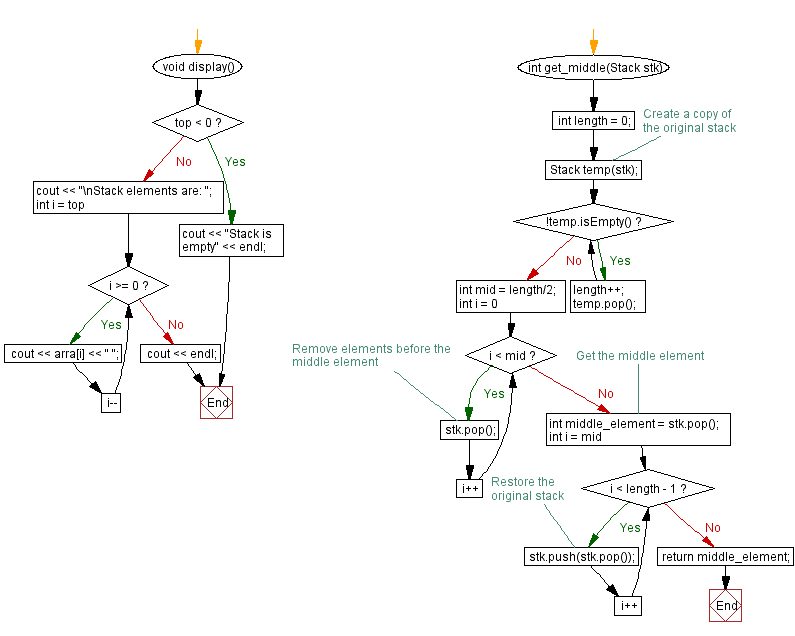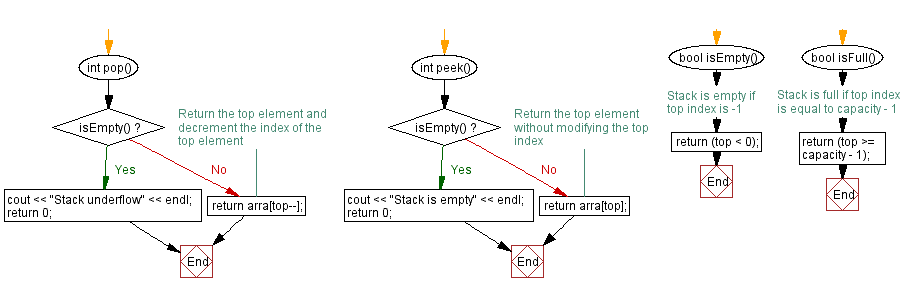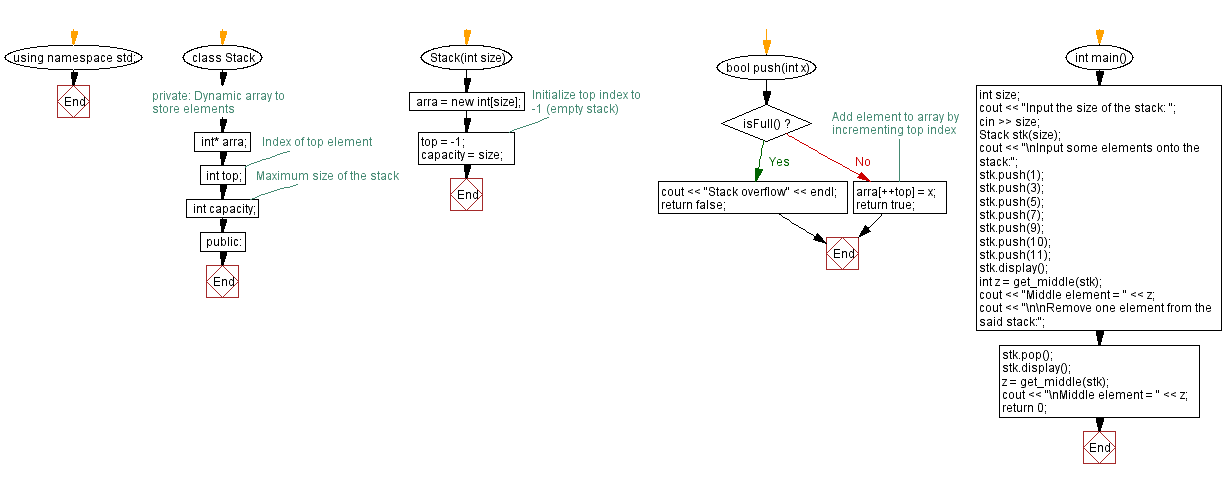﻿ C++ Middle element of a stack (using a dynamic array)

# C++ Stack Exercises: Middle element of a stack (using a dynamic array)

## C++ Stack: Exercise-23 with Solution

Write a C++ program to find the middle element of a stack (using a dynamic array).

Test Data:
Input the size of the stack: 8
Input some elements onto the stack:
Stack elements are: 4 7 5 3 1
Reverse the said stack elements:
Stack elements are: 1 3 5 7 4

Sample Solution:

C++ Code:

``````#include <iostream>

using namespace std;

class Stack {
private:
// Dynamic array to store elements
int* arra;
int top;        // Index of top element
int capacity;   // Maximum size of the stack
public:
Stack(int size) {
arra = new int[size];
top = -1;       // Initialize top index to -1 (empty stack)
capacity = size;
}

bool push(int x) {
if (isFull()) {
cout << "Stack overflow" << endl;
return false;
}
//Add element to array by incrementing top index
arra[++top] = x;
return true;
}

int pop() {
if (isEmpty()) {
cout << "Stack underflow" << endl;
return 0;
}
//Return the top element and decrement the index of the top element
return arra[top--];
}

int peek() {
if (isEmpty()) {
cout << "Stack is empty" << endl;
return 0;
}
// Return the top element without modifying the top index
return arra[top];
}

bool isEmpty() {
// Stack is empty if top index is -1
return (top < 0);
}

bool isFull() {
// Stack is full if top index is equal to capacity - 1
return (top >= capacity - 1);
}

void display() {
if (top < 0) {
cout << "Stack is empty" << endl;
return;
}
cout << "\nStack elements are: ";
for (int i = top; i >= 0; i--)
cout << arra[i] << " ";
cout << endl;
}
};

int get_middle(Stack stk) {
int length = 0;
// Create a copy of the original stack
Stack temp(stk);
while (!temp.isEmpty()) {
length++;
temp.pop();
}
int mid = length/2;
for (int i = 0; i < mid; i++) {
// Remove elements before the middle element
stk.pop();
}
// Get the middle element
int middle_element = stk.pop();
for (int i = mid; i < length - 1; i++) {
// Restore the original stack
stk.push(stk.pop());
}
return middle_element;
}
int main() {
int size;
cout << "Input the size of the stack: ";
cin >> size;
Stack stk(size);
cout << "\nInput some elements onto the stack:";
stk.push(1);
stk.push(3);
stk.push(5);
stk.push(7);
stk.push(9);
stk.push(10);
stk.push(11);
stk.display();
int z = get_middle(stk);
cout << "Middle element = " << z;
cout << "\n\nRemove one element from the said stack:";
stk.pop();
stk.display();
z = get_middle(stk);
cout << "\nMiddle element = " << z;
return 0;
}
``````

Sample Output:

```Input the size of the stack: 8

Input some elements onto the stack:
Stack elements are: 11 10 9 7 5 3 1
Middle element = 7

Remove one element from the said stack:
Stack elements are: 10 9 7 5 3 1

Middle element = 5
```

Flowchart:CPP Code Editor: